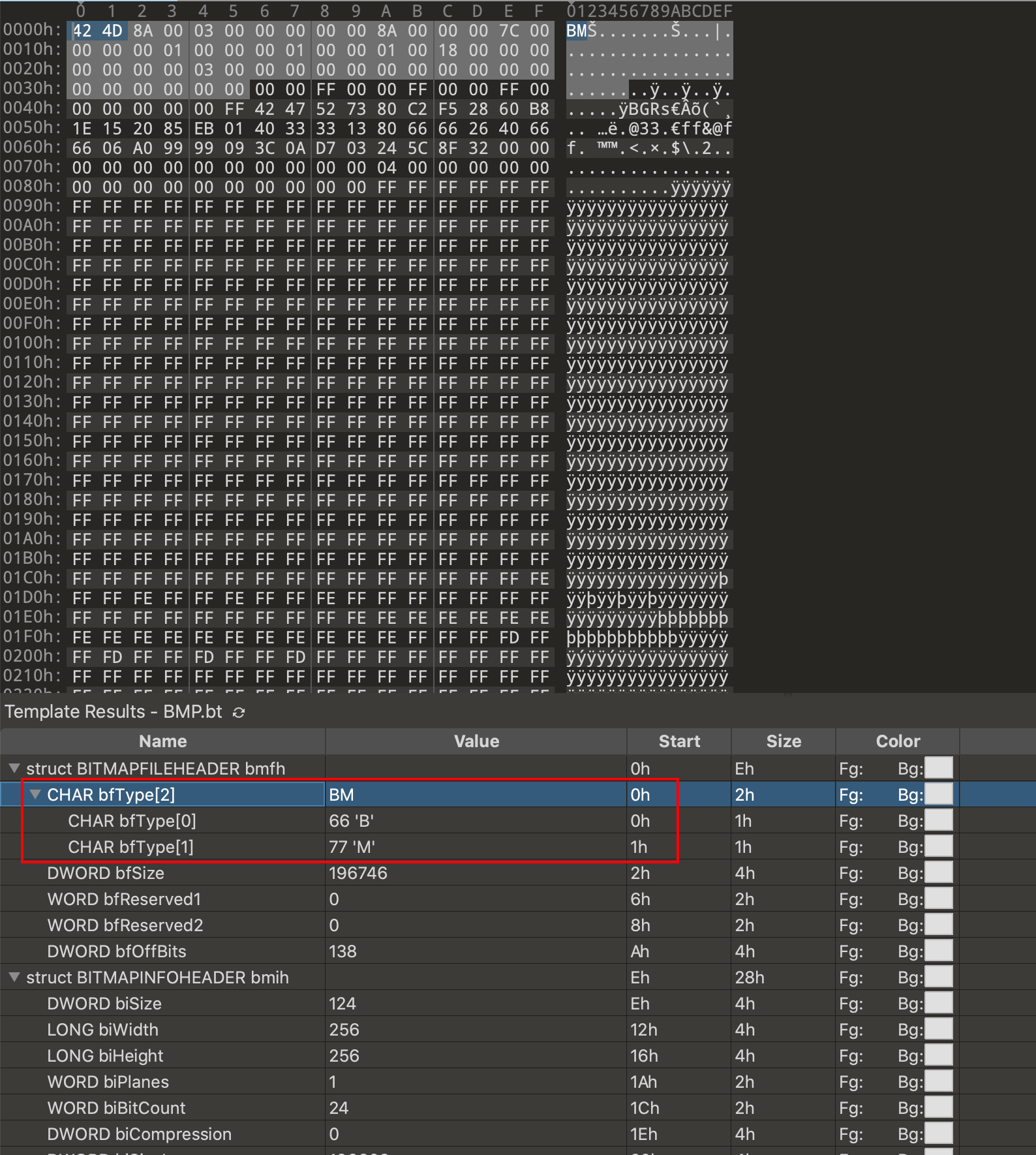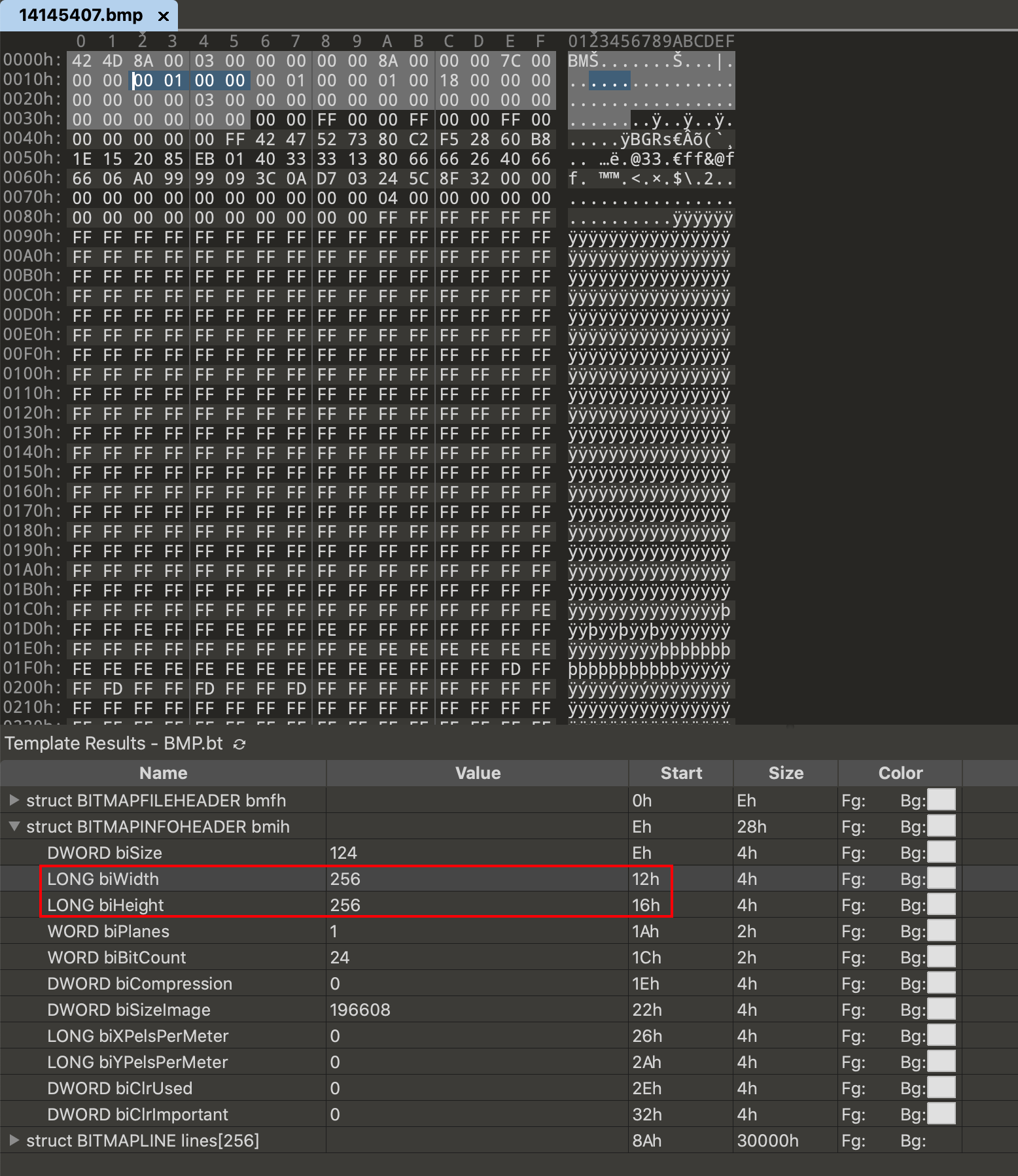# image_size_getter 2.1.2image_size_getter: ^2.1.2 copied to clipboard

Get image width and height, the library does not completely decode the image file, just read the metadata to get the image width and height.

# image_size_getter #

Do not completely decode the image file, just read the metadata to get the image width and height.

Just support jpeg, gif, png, webp, bmp.

## Usage #

### Image of File #

``````import 'dart:io';

import 'package:image_size_getter/image_size_getter.dart';
import 'package:image_size_getter/file_input.dart'; // For compatibility with flutter web.

void main(List<String> arguments) async {
final file = File('asset/IMG_20180908_080245.jpg');
final size = ImageSizeGetter.getSize(FileInput(file));
print('jpg = \$size');

final pngFile = File('asset/ic_launcher.png');
final pngSize = ImageSizeGetter.getSize(FileInput(pngFile));
print('png = \$pngSize');

final webpFile = File('asset/demo.webp');
final webpSize = ImageSizeGetter.getSize(FileInput(webpFile));
print('webp = \$webpSize');

final gifFile = File('asset/dialog.gif');
final gifSize = ImageSizeGetter.getSize(FileInput(gifFile));
print('gif = \$gifSize');
}

``````

### Image of Memory #

``````import 'package:image_size_getter/image_size_getter.dart';

void foo(Uint8List image){
final memoryImageSize = ImageSizeGetter.getSize(MemoryInput(image));
print('memoryImageSize = \$memoryImageSize');
}
``````

### Image of Http #

See HttpInput.

The size contains width and height.

But some image have orientation. Such as jpeg, when the orientation of exif is 5, 6, 7 or 8, the width and height will be swapped.

We can use next code to get width and height.

``````void foo(File file) {
final size = ImageSizeGetter.getSize(FileInput(file));
if (size.needRotate) {
final width = size.height;
final height = size.width;
print('width = \$width, height = \$height');
} else {
print('width = \${size.width}, height = \${size.height}');
}
}
``````

## AsyncImageInput #

If your data source is read asynchronously, consider using `AsyncImageInput`.

A typical use case is http_input.

## Custom #

We can implement our own input or decoder.

In addition to several built-in implementations, subsequent implementations will also be added to the project through plugin.

### Custom Input #

Such as: http_input.

In addition, if your picture has verification, for example, you need to use the request header to access it, or you need a post request to get it, you need to customize input.

### Custom Decoder #

Such as bmp decoder

Check the file type:The width and height:So, we can write code with:

``````import 'package:image_size_getter/image_size_getter.dart';

class BmpDecoder extends BaseDecoder {
const BmpDecoder();

@override
String get decoderName => 'bmp';

@override
Size getSize(ImageInput input) {
final widthList = input.getRange(0x12, 0x16);
final heightList = input.getRange(0x16, 0x1a);

final width = convertRadix16ToInt(widthList, reverse: true);
final height = convertRadix16ToInt(heightList, reverse: true);
return Size(width, height);
}

@override
Future<Size> getSizeAsync(AsyncImageInput input) async {
final widthList = await input.getRange(0x12, 0x16);
final heightList = await input.getRange(0x16, 0x1a);

final width = convertRadix16ToInt(widthList, reverse: true);
final height = convertRadix16ToInt(heightList, reverse: true);
return Size(width, height);
}

@override
bool isValid(ImageInput input) {
final list = input.getRange(0, 2);
return _isBmp(list);
}

@override
Future<bool> isValidAsync(AsyncImageInput input) async {
final list = await input.getRange(0, 2);
return _isBmp(list);
}

bool _isBmp(List<int> startList) {
return startList == 66 && startList == 77;
}
}

``````

Use it:

``````final bmp = File('../example/asset/demo.bmp');

const BmpDecoder decoder = BmpDecoder();
final input = FileInput(bmp);

assert(decoder.isValid(input));
expect(decoder.getSize(input), Size(256, 256));
``````

#### Register custom decoder to image size getter

``````ImageSizeGetter.registerDecoder(const BmpDecoder());
``````

The method can also be used to replace the default decoder.

For example, you think the existing JPEG format is not rigorous enough.

``````ImageSizeGetter.registerDecoder(const MyJpegDecoder());
``````

#### Use decoder alone

Each decoder can be used alone.

``````void decodeWithImageInput(ImageInput input) {
BaseDecoder decoder = const GifDecoder();
final isGif = decoder.isValid(input);
print('isGif: \$isGif');

if (isGif) {
final size = decoder.getSize(input);
print('size: \$size');
}
}

void decodeWithAsyncImageInput(AsyncImageInput input) async {
BaseDecoder decoder = const PngDecoder();
final isPng = await decoder.isValidAsync(input);
print('isPng: \$isPng');

if (isPng) {
final size = await decoder.getSizeAsync(input);
print('size: \$size');
}
}
``````

See migrate

## Other question #

The package is dart package, no just flutter package. So, if you want to get flutter asset image size, you must convert it to memory(Uint8List).

``````final buffer = await rootBundle.load('assets/logo.png'); // get the byte buffer
final memoryImageSize = ImageSizeGetter.getSize(MemoryInput.byteBuffer(buffer));
print('memoryImageSize = \$memoryImageSize');
``````

Apache 2.0

78
likes
130
pub points
97%
popularity

### Publisherkikt.top

Get image width and height, the library does not completely decode the image file, just read the metadata to get the image width and height.

### DocumentationApache-2.0 (LICENSE)